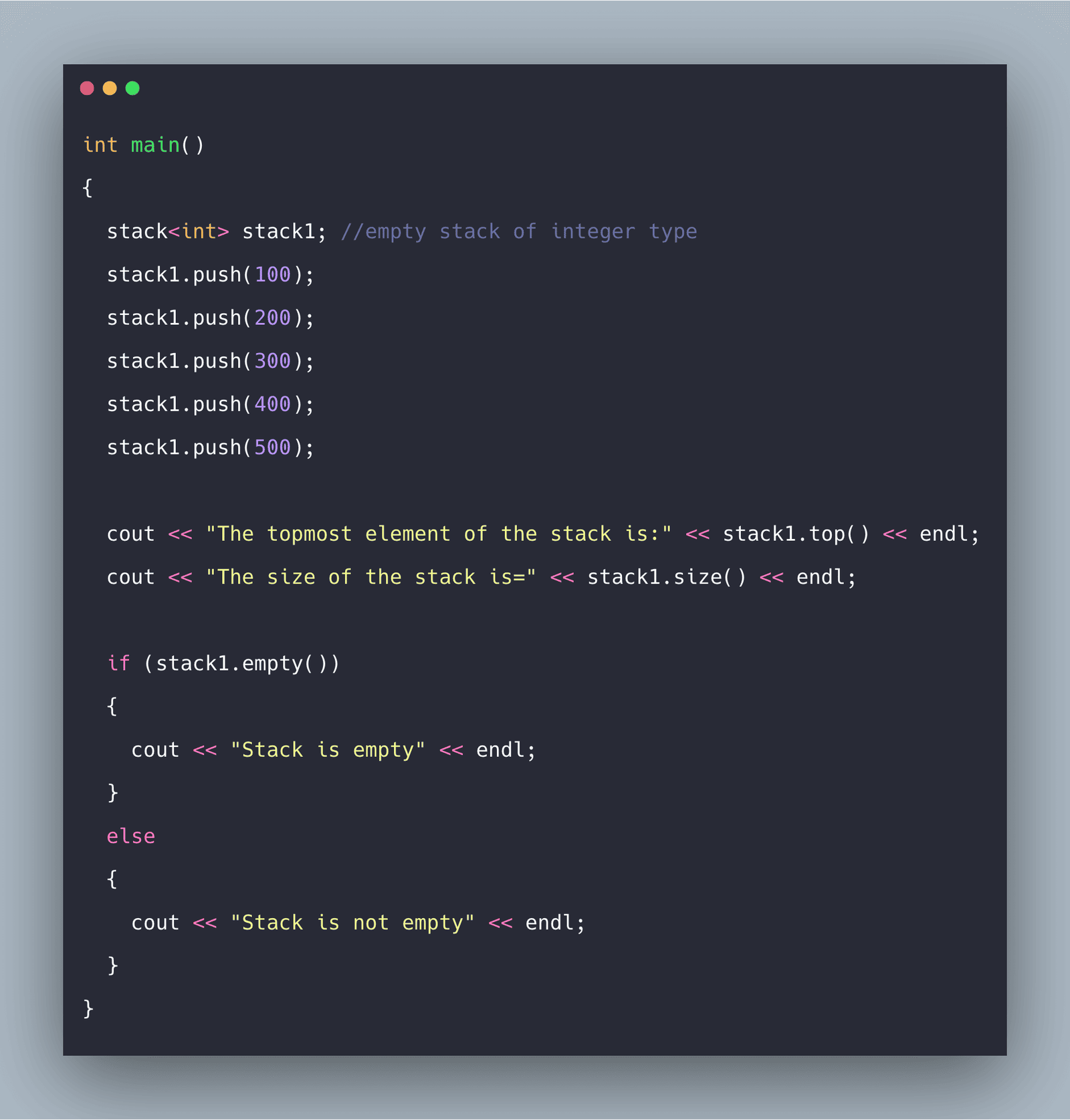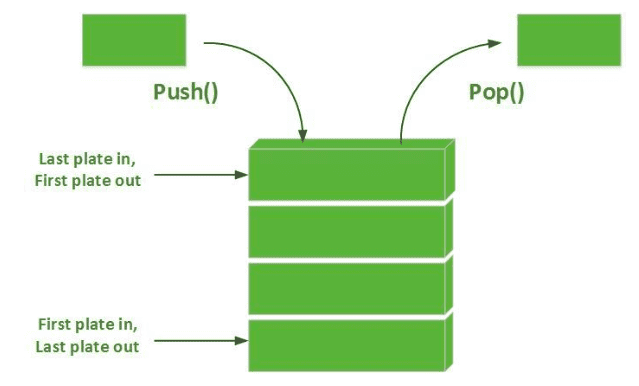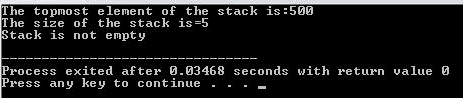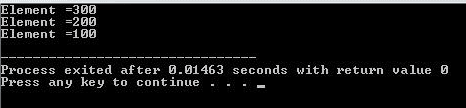# Stack in C++: The Complete Guide

0
971The stack is a data structure that works on the principle of LIFO(Last in, first out). In stacks, we insert elements from one side and only remove the items from that side. The stack is a container adapter that uses an encapsulated object of a specific container class, which provides a particular set of member functions to access its elements.

## Stack in C++

Stacks in C++ are a container adaptor with LIFO(Last In First Out) type of work, where the new element is added at one end and (top) an item is removed from that end only. In the stack data structure, the elements inserted initially are taken out from the stack at last.

We can use stacks in PDA(Pushdown Automata).Header file required to use stack in C++ – #include<stack>

With this we can use stack STL.

## Different functions associated with stacks

### empty()

The empty() function returns whether the stack is empty or not.

#### Syntax

`stack_name.empty()`

We don’t pass any parameter, and it returns true if the stack is empty or false otherwise.

#### Example

```stack1 = 1,2,3

stack1.empty();```

```False
```

### size()

It returns several items in the stack.

#### Syntax

```stack_name.size()
```

We don’t pass any parameter in this, and it returns the number of elements in the stack container.

#### Example

```stack_1 = 1,2,3,4,5
stack_1.size();
```

`5`

### top()

It returns a reference to the topmost element of a stack.

#### Syntax

```stack_name.top();
```

We don’t need to pass any parameter, and it returns a direct reference of the top element.

#### Example

```stack_name.push(5);
stack_name.push(6);
stack_name.top();
```

`6`

### push(k)

The push() function is used to insert the elements in the stack.

It adds the element ‘k’ at the top of the stack.

#### Syntax

```stack_name.push(value)
```

In this, we pass the value as a parameter and, as a result, add the element to the stack.

#### Example

```stack1.push(77)
stack1.push(88)
```

#### Output

```77, 88
```

### pop()

It deletes the topmost element from the stack.

#### Syntax

```stack_name.pop()
```

In this, we don’t pass any parameters. This function pops the topmost element from the stack.

#### Example

```stack1 = 10,20,30;
stack1.pop();```

`10, 20`

#### Errors and Exceptions

1. Shows error if a parameter is passed.
2. Shows no exception throw guarantee.

## C++ Stack Algorithm

In stack-related algorithms, the TOP initially points to 0, the index of elements in the stack starts from 1, and an index of the last element is MAX.

```    INIT_STACK (STACK, TOP)

Algorithm to initialize a stack using array.
TOP points to the top-most element of stack.

1) TOP: = 0;
2) Exit
```

#### The push() operation is used to insert an element into the stack.

```    PUSH_STACK(STACK,TOP,MAX,ITEM)

Algorithm to push an item into stack.

1) IF TOP = MAX   then
Print “Stack is full”;
Exit;
2) Otherwise
TOP: = TOP + 1;        /*increment TOP*/
STACK (TOP):= ITEM;
3) End of IF
4) Exit
```

#### The pop() operation is used to delete the item from the stack, get an item and then decrease the TOP pointer.

```    POP_STACK(STACK,TOP,ITEM)

Algorithm to pop an element from stack.

1) IF TOP = 0 then
Print “Stack is empty”;
Exit;
2) Otherwise
ITEM: =STACK (TOP);
TOP:=TOP – 1;
3) End of IF
4) Exit
```
```    IS_FULL(STACK,TOP,MAX,STATUS)

Algorithm to check stack is full or not.
STATUS contains the result status.

1) IF TOP = MAX then
STATUS:=true;
2) Otherwise
STATUS:=false;
3)  End of IF
4)  Exit
```
```    IS_EMPTY(STACK,TOP,MAX,STATUS)

Algorithm to check stack is empty or not.
STATUS contains the result status.

1) IF TOP = 0 then
STATUS:=true;
2) Otherwise
STATUS:=false;
3)  End of IF
4)  Exit```

## C++ Stack Program

Q1- Write a program to insert five elements in the stack and print the top element using top() and print the size of the stack and check if the stack is empty or not.

```#include <iostream>
#include <stack>

using namespace std;

int main()
{
stack<int> stack1; //empty stack of integer type
stack1.push(100);
stack1.push(200);
stack1.push(300);
stack1.push(400);
stack1.push(500);

cout << "The topmost element of the stack is:" << stack1.top() << endl;
cout << "The size of the stack is=" << stack1.size() << endl;

if (stack1.empty())
{
cout << "Stack is empty" << endl;
}
else
{
cout << "Stack is not empty" << endl;
}
}```

See the output.Q2- Write a program to insert 5 elements in a stack, delete 2 elements, and print the stack.

```#include <iostream>
#include <stack>

using namespace std;

int main()
{
stack<int> stack1; //empty stack of integer type
stack1.push(100);
stack1.push(200);
stack1.push(300);
stack1.push(400);
stack1.push(500);

stack1.pop();
stack1.pop();

while (!stack1.empty())
{
cout << "Element =" << stack1.top() << endl;
stack1.pop();
}
}```

See the output.## Applications of Stack

1. Conversion of polish notations
There are three types of notations:
1) Infix notation – An Operator is between the operands: x + y
2) Prefix notation – An Operator is before the operands: + xy
3) Postfix notation – An Operator is after the operands: xy +
2. To reverse a string
A string can be reversed by using the stack. First, the string characters are pushed onto the stack till the end of the string. Then, the characters are popped and displayed. Since the end character of the string is pushed, at last, it will be printed first.
3. When a function (sub-program) is called
When the function is called, the last function will be completed first. It is the property of the stack. There is a memory area specifically reserved for the stack.

## Conclusion

Stack is an abstract data structure that contains a collection of elements.

Stack implements the LIFO mechanism, i.e., the element that is pushed at the end is popped out first.

That’s it.

## Recommended Posts

Priority Queue in C++

C++ Queue Standard Template Library

C++ Deque

Arrays in C++

Iterators in C++

This site uses Akismet to reduce spam. Learn how your comment data is processed.